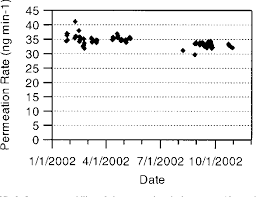## How to Calculate and Solve for Permeation | Mass TransferThe image above represents permeation.

To compute for permeation, six essential parameters are needed and these parameters are Length of Vessel (L), Diameter (D), Initial Concentration (c1), Final Concentration (c2), Inlet Radius of Pipe (r1and Exit Radius of Pipe (r2).

The formula for calculating permeation:

j = -2πLD (c1 – c2 / In(r1/r2))

Where:

j = Permeation
L = Length of Vessel
D = Diameter
c1 = Initial Concentration
c2 = Final Concentration
r1 = Inlet Radius of Pipe
r2 = Exit Radius of Pipe

Let’s solve an example;
Find the permeation when the length of vessel is 8, the diameter is 2, the initial concentration is 4, the final concentration is 9, the inlet radius of pipe is 13 and the exit radius of pipe is 11.

This implies that;

L = Length of Vessel = 8
D = Diameter = 2
c1 = Initial Concentration = 4
c2 = Final Concentration = 9
r1 = Inlet Radius of Pipe = 13
r2 = Exit Radius of Pipe = 11

j = -2πLD (c1 – c2 / In(r1/r2))
j = -2π(8)(2) (4 – 9/In(13/11))
j = (-100.53) (-5/In(1.18))
j = (-100.53) (-5/0.167)
j = (-100.53) (-29.93)
j = 3008.9

Therefore, the permeation is 3008.9 m².